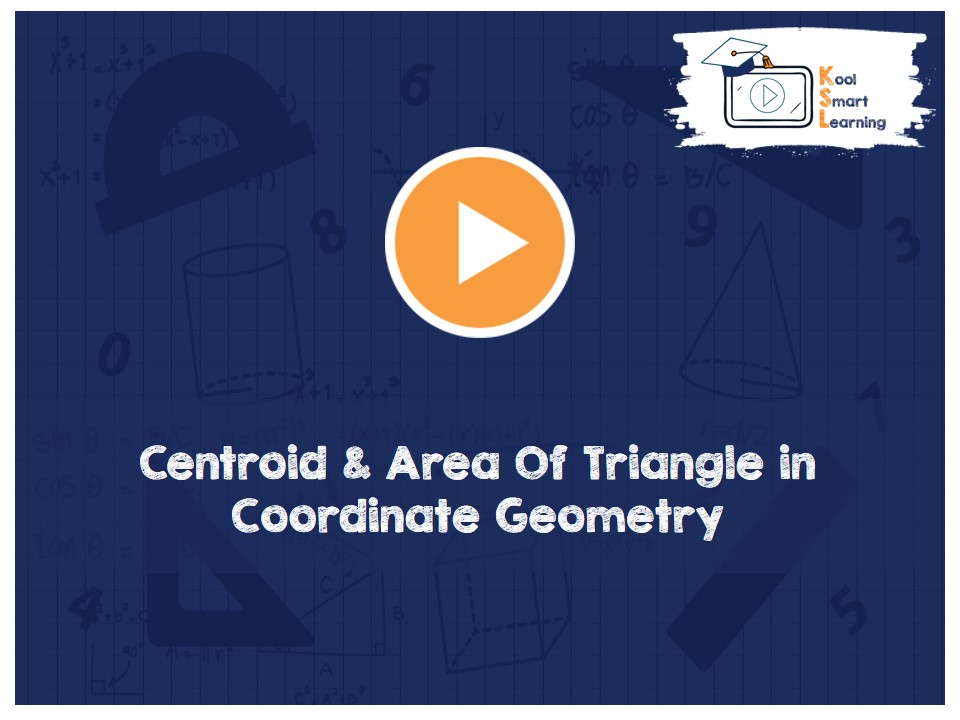At KoolSmartLearning, we intend to harness the power of online education to make learning easy.## Centroid & Area Of Triangle in Coordinate Geometry

/  Centroid & Area Of Triangle in Coordinate Geometry## Centroid & Area Of Triangle in Coordinate Geometry

The video discusses the formula for finding the centroid using the section formula if the co-ordinates of its vertices are given. It also explains the formula of calculating the area of the triangle, along with the derivation of the same, if the co-ordinates of its vertices are given.

More Related Videos